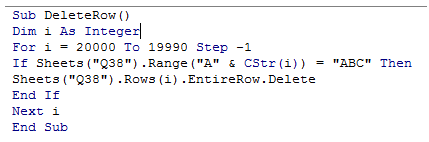## Vba delete rows with vba in Excel

What is it with deleting a row in VBA? Is that too much difficult? Why is this a separate chapter?

Because, Deleting a row may be a simple task, but there is a difficulty.

So, If Row 5 has to be deleted from a sheet, it will be a simple one line code, say for example, Rows(5).Delete.

Consider a situation, where a row has to be deleted based on a value in a particular cell, say for example,

delete a row if there is "ABC" in Cell A5, and there are 40 rows in total.

The simplest and only way is to loop through A and once the value is found, delete that row, that is, Rows(5).Delete.

Here rises the issue, once Row(5) is deleted, the Row6 becomes, Row5 and if there is the same value there too, that is "ABC",

then, this row will not be deleted as it is Row5 and the program is on Row 6.

Hence, while deleting Rows in VBA, The loop should be from the last used Row and upwards.

By doing so, the the Rows does not shift after deleting and data is preserved.

The following example illustrates this:

1. Sub DeleteRow()
2. Dim i As Integer
3. For i = 20000 To 19990 Step -1
4. If Sheets("Q38").Range("A" & CStr(i)) = "ABC" Then
5. Sheets("Q38").Rows(i).EntireRow.Delete
6. End If
7. Next i
8. End Sub

The screenshot of the editor is shown below:Note that, we have used Rows 19990 to 20000 just for illustration since it will not affect the layout of this sheet.